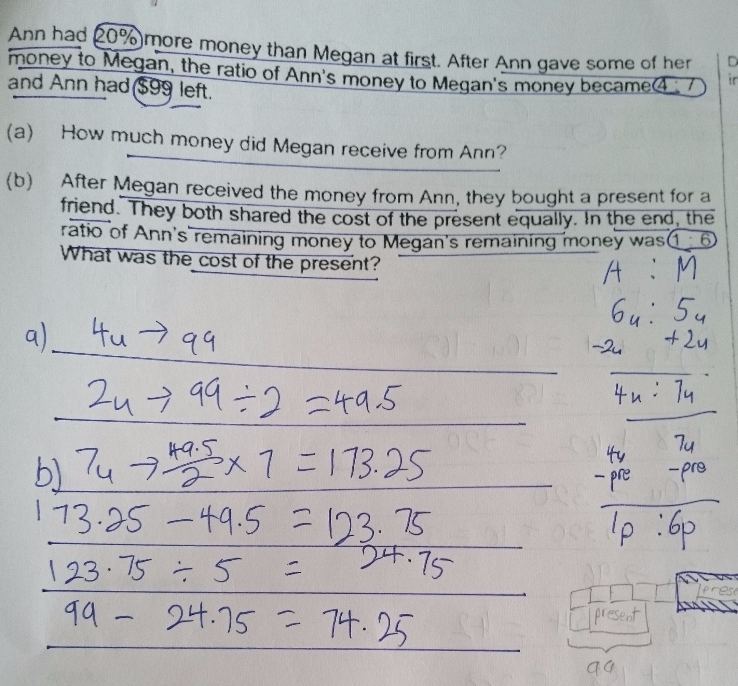QuestionHi, please let me know why answer for (b) is wrong.  Thank you.

Source: Henry Park Primary

second step should be 173.25 – 99 = 74.25

(or you could find 3 units instead of 7 units)

Oops! didn’t think of that.

Thank you for pointing out dazzlego.

0 Replies 0 Likes

This is a Before After question.

 After Transfer Before Ann 99 + 1u 99 + 1u Megan 173.25 – 1u 173.25 – 1u

4u = 99

1u = 49 ÷ 4 = 24.75

7u = 7 x 24.75 = 173.25

a)

(99 + 1u) / 120 = (173.25 – 1u) / 100

(99 + 1u) / 6 = (173.25 – 1u) / 5

495 + 5u = 1038.5 – 6u

5u + 6u = 1039.5 – 495

11u = 544.5

1u = 544.5 ÷ 11 = 49.5

b)

(99 – 1b) / 1 = (173.25 – 1b) / 6

594 – 6b = 173.25 – 1b

594 – 173.25 = 6b – 1b

5b = 420.75

1b = 420.75 ÷ 5 = 84.15

2b = 2 x 84.15 = \$168.30

Thank you Mr Tay and Mr Khong for further options.

0 Replies 0 Likes

Method 1 :
(a)
At first —
Ann : 1.2u
Megan : 1u

After —
Ann : (4/11) x 2.2 = 0.8u ——- 99
Megan : (7/11) x 2.2 = 1.4u

1u ——- 99/0.8 = 123.75
1.4u – 1u = 0.4u ——- 0.4 x 123.75 = 49.50

(b)
cost of the present ——- 2p
(0.8u – 1p) x 6 = 4.8u – 6p ——- 1.4u – 1p
1p ——- (4.8u – 1.4u)/5 = 0.68u = 0.68 x 123.75 = 84.15
2p ——- 2 x 84.15 = 168.30

Method 2 :
(a)
At first —
Ann : Megan : Total
12u : 10u : 22u

After (Ann Gave some of her money to Megan,) —
Ann : Megan : Total
4 : 7 : 11
8u : 14u : 22u

8u ——- 99
14u – 10u = 4u ——- 99/2 = 49.50

(b)
In the end —
Ann : Megan
1p : 6p

8u – 1p ——- 14u – 6p
5p ——- 6u
1u ——- 49.50/4 = 12.375
6u ——- 6 x 12.375 = 74.25
1p ——– 74.25/5 = 14.85
(8u – 1p) x 2 ——- (8 x 12.375 – 14.85) x 2 = 168.30
or
(14u – 6p) x 2 ——– (14 x 12.375 – 6 x 14.85) x 2 = 168.30

Ans : (a) \$48.50; (b) \$168.30.

0 Replies 1 Like

This is a Before After question.

 After Transfer Before Ann 99 + 1u 99 + 1u Megan 173.25 – 1u 173.25 – 1u

4u = 99

1u = 49 ÷ 4 = 24.75

7u = 7 x 24.75 = 173.25

a)

(99 + 1u) / 120 = (173.25 – 1u) / 100

(99 + 1u) / 6 = (173.25 – 1u) / 5

495 + 5u = 1038.5 – 6u

5u + 6u = 1039.5 – 495

11u = 544.5

1u = 544.5 ÷ 11 = 49.5

b)

(99 – 1b) / 1 = (173.25 – 1b) / 6

594 – 6b = 173.25 – 1b

594 – 173.25 = 6b – 1b

5b = 420.75

1b = 420.75 ÷ 5 = 84.15

2b = 2 x 84.15 = \$168.30

0 Replies 0 Likes